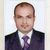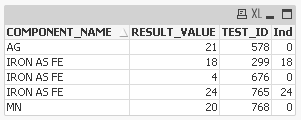Announcements
You can succeed best and quickest by helping others to succeed. Join the conversation.
cancel
Showing results for
Did you mean:Creator III

## if condition not working properly

Hi everyone,

I am trying to run a query,but I am not getting the desired output.

COMPONENT_NAME,

RESULT_VALUE,

TEST_ID,

if(upper(COMPONENT_NAME)='IRON AS FE',Count(if(RESULT_VALUE >=15,'5'))) as Ind

from result.qvd

Group by

COMPONENT_NAME,RESULT_VALUE,TEST_ID;

But everytime it get 0,1 as Ind

Is there any pblm ion my script???

1 Solution

Accepted SolutionsCreator III
Author

Hi All,

I found the solution for this.

Final:

COMPONENT_NAME,

RESULT_VALUE,

if(upper(COMPONENT_NAME)='IRON AS FE' and RESULT_VALUE >=15,RESULT_VALUE)  as Ind

FROM

[RESULT.qvd]

(qvd);

temp:

Resident Final Where Exists (Ind);

let v_var=NoOfRows('temp');

load distinct *,'\$(v_var)' as Count resident Final;

Here we can get the count at backend only.

13 RepliesMVP

Your count expression is wrong in the load script that required the field name there

In this below line you have error in place of '5' you need field name

if(upper(COMPONENT_NAME)='IRON AS FE',Count(if(RESULT_VALUE >=15,'5'))) as Ind

That may be

if(upper(COMPONENT_NAME)='IRON AS FE',Count(if(RESULT_VALUE >=15,FieldName))) as Ind

If possible share the sample file.Creator III
Author

Hi,

The above given solution doesn't works.

my data is something like

COMPONENT_NAME     RESULT_VALUE    TEST_ID

IRON AS FE                    18                         299

MN                                  20                          768

AG                                   21                         578

IRON AS FE                    24                         765

IRON AS FE                    4                              676

I want to calculate only those values whosename is IRON AS FE and count is above 15 and name it as IND.The query I am using is.

COMPONENT_NAME,

RESULT_VALUE,

TEST_ID,

if(upper(COMPONENT_NAME)='IRON AS FE',Count(if(RESULT_VALUE >=15,RESULT_VALUE ,))) as Ind

from result.qvd

Group by

COMPONENT_NAME,RESULT_VALUE,TEST_ID;

But I am getting oly 0,1 as IND.MVP

Update:- Now check i make some changes remove one dummy row

Source:

COMPONENT_NAME, RESULT_VALUE, TEST_ID

IRON AS FE, 18, 299

MN, 20, 768

AG, 21, 578

IRON AS FE, 24, 765

IRON AS FE, 4, 676

];

Final:

COMPONENT_NAME,

RESULT_VALUE,

TEST_ID,

if(upper(COMPONENT_NAME) = 'IRON AS FE' and RESULT_VALUE >= 15,Count(TEST_ID)) as Ind

Resident Source

Group by

COMPONENT_NAME,RESULT_VALUE,TEST_ID;

DROP Table Source;

Note:- But my question is why you Count the TEST_ID because it will gives you 1 for the TEST_ID if it is present think about this or may be you want to Sum the TEST_ID values then use like below

if(upper(COMPONENT_NAME) = 'IRON AS FE' and RESULT_VALUE >= 15,Sum(TEST_ID)) as Ind2Creator III
Author

Hi,

I don't want to count the test values,rather I need the values of result_value whose value is greater then 15.I further need these values for further calculations.MVP

Ok then you have to use below script in load script replace with this

if(upper(COMPONENT_NAME) = 'IRON AS FE' and RESULT_VALUE >= 15,RESULT_VALUE) as Ind

And let me know what is your expected out put from the sample data and why you use TEST_ID.Creator III
Author

I tried this,but it is not providing the correct outputMVP

What is your expected out put from when you add below script

if(upper(COMPONENT_NAME) = 'IRON AS FE' and RESULT_VALUE >= 15,RESULT_VALUE,0) as Ind

You get the below out put

for Ind field >>Creator III
Author

FINAL_RESULT:

COMPONENT_NAME,

RESULT_VALUE,

TEST_ID,

if(upper(COMPONENT_NAME) = 'IRON AS FE' and RESULT_VALUE >= 15,RESULT_VALUE) as Ind

FROM RESULT.qvd;

//Group by

//COMPONENT_NAME,RESULT_VALUE,TEST_ID;

exit Script;

//DROP TABLE RESULT;

It is working fine,But I want the count of RESULT_VALUE whose value >15  as Ind.

I am getting the values,but I only need the count not the values.MVP

Then you have to load a table same and add new field with count and aggregate the data see the script

NAL_RESULT:

COMPONENT_NAME,

RESULT_VALUE,

TEST_ID,

if(upper(COMPONENT_NAME) = 'IRON AS FE' and RESULT_VALUE >= 15,RESULT_VALUE) as Ind,

Count(if(upper(COMPONENT_NAME) = 'IRON AS FE' and RESULT_VALUE >= 15,RESULT_VALUE)) as ResultCount

FROM RESULT.qvd;

Group by

COMPONENT_NAME,RESULT_VALUE,TEST_ID;

Exit Script;

DROP TABLE RESULT;

And then in front end Straight table chart

Dimension:- COMPONENT_NAME

Expr:- Sum(ResultCount)

For calculate the values or you can load another table also

Details: